×#### Thank you for registering.

One of our academic counsellors will contact you within 1 working day.

Click to Chat

1800-1023-196

+91-120-4616500

CART 0

• 0

MY CART (5)

Use Coupon: CART20 and get 20% off on all online Study Material

ITEM
DETAILS
MRP
DISCOUNT
FINAL PRICE
Total Price: Rs.

There are no items in this cart.
Continue Shopping• Complete JEE Main/Advanced Course and Test Series
• OFFERED PRICE: Rs. 15,900
• View Details

Chapter 20: Linear Equation in One Variable Exercise – 20.1

Question: 1

A flooring tile has the shape of a parallelogram whose base is 24 cm and the corresponding height is 10 cm. How many are such tiles required to cover a floor of area 1080 m2?

Solution:

Given: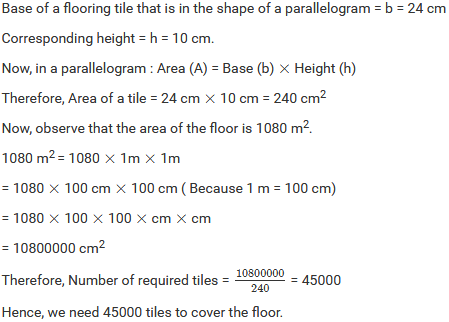Question: 2

A plot is in the form of a rectangle ABCD having semi-circle on BC as shown in Fig. 20.23. If AB = 60 m and BC = 28 m, find the area of the plot.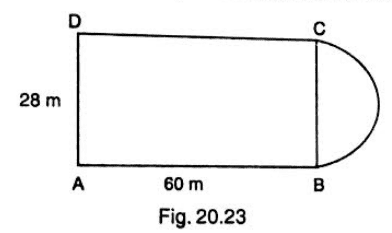Solution: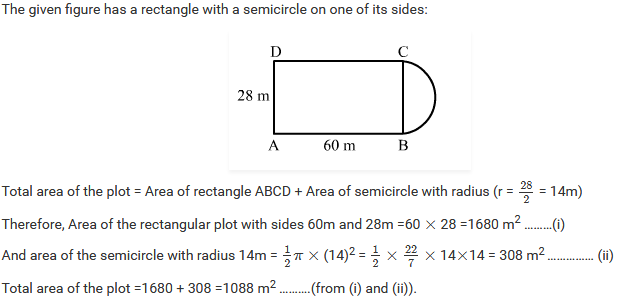Question: 3

A playground has the shape of a rectangle, with two semi-circles on its smaller sides as diameters, added to its outside. If the sides of the rectangle are 36 m and 24.5 m, find the area of the playground. (Take pi = 22 / 7 ).

Solution: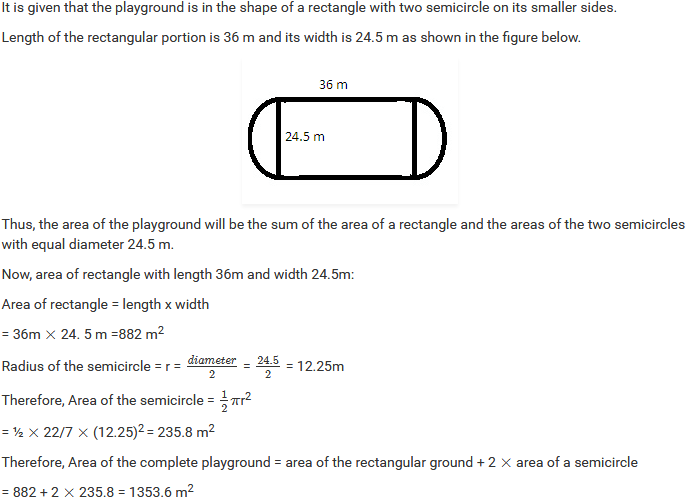Question: 4

A rectangular piece is 20 m long and 15 m wide. From its four corners, quadrants of radii 3.5 m have been cut. Find the area of the remaining part.

Solution: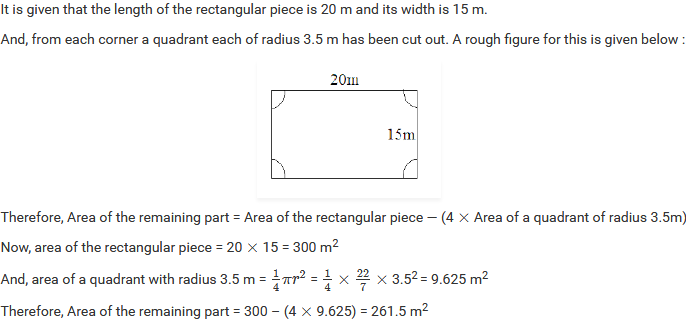Question: 5

The inside perimeter of a running track (shown in Fig. 20.24) is 400 m. The length of each of the straight portion is 90 m and the ends are semi-circles. If the track is everywhere 14 m wide, find the area of the track. Also, find the length of the outer running track.

Solution:It is given that the inside perimeter of the running track is 400m. It means the length of the inner track is 400 m.

Let r be the radius of the inner semicircles.

Observe: Perimeter of the inner track = Length of two straight portions of 90 m + Length of two semicircles 400

Therefore, 400 = (2 × 90) + (2 × Perimeter of a semicircle)

400 = 180 + (2 × 22/7 × r)

400 -180 = (44/7 × r)

44/7 × r = 220Question: 6

Find the area of Fig. below, in square cm, correct to one place of decimal. (Take it = 22/7)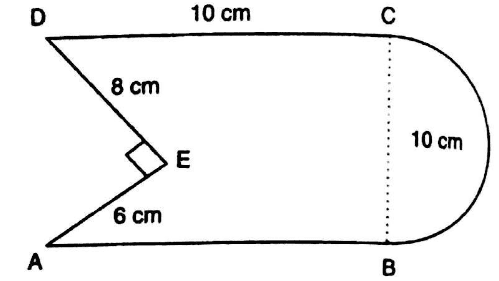Solution:

The given figure is: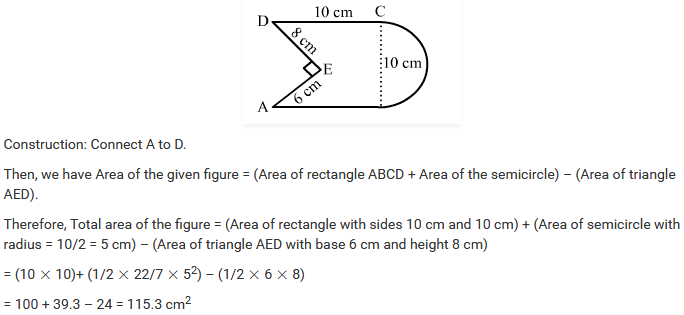Question: 7

The diameter of a wheel of a bus is 90 cm which makes 315 revolutions per minute. Determine its speed in kilometers per hour. [Use pi = 22 / 7].

Solution:Question: 8

The area of a rhombus is 240 cm2 and one of the diagonal is 16 cm. Find another diagonal.

Solution: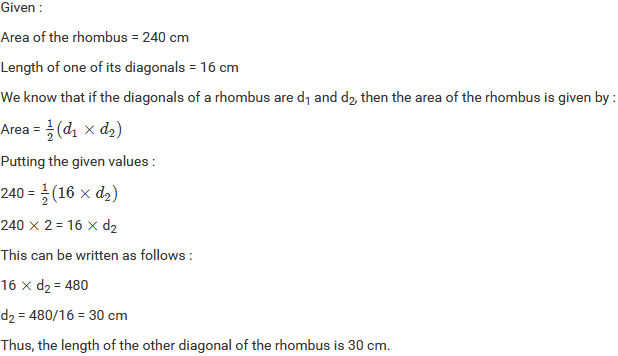Question: 9

The diagonals of a rhombus are 7.5 cm and 12 cm. Find its area.

Solution:Question: 10

The diagonal of a quadrilateral shaped field is 24 m and the perpendiculars dropped on it from the remaining opposite vertices are 8 m and 13 m. find the area of the field.

Solution:Question: 11

Find the area of a rhombus whose side is 6 cm and whose altitude is 4 cm. If one of its diagonals is 8 cm long, find the length of the other diagonal.

Solution: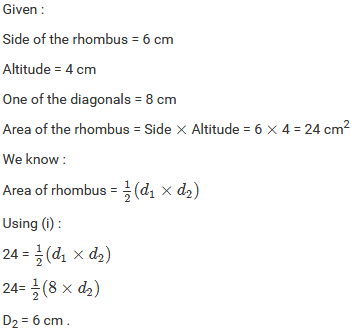Question: 12

The floor of a building consists of 3000 tiles which are rhombus shaped and each of its diagonals are 45 cm and 30 cm in length. Find the total cost of polishing the floor, if the cost per m2 is Rs 4.

Solution: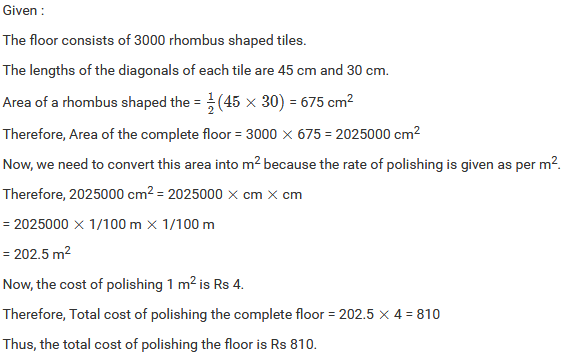Question: 13

A rectangular grassy plot is 112 m long and 78 m broad. It has a gravel path 2.5 m wide all around it on the side. Find the area of the path and the cost of constructing it at Rs 4.50 per square meter.

Solution: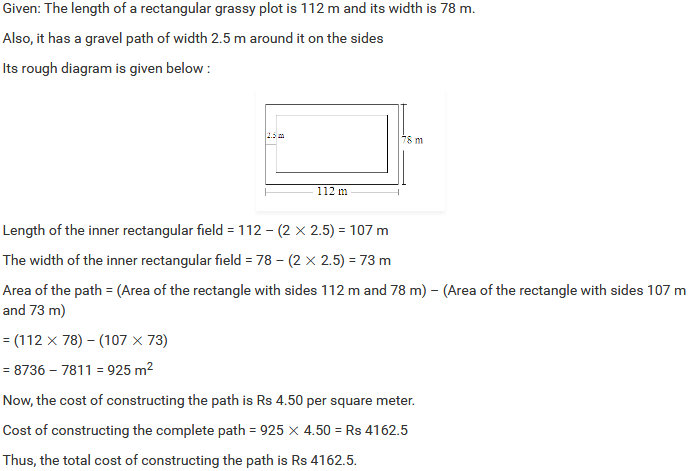Question: 14

Find the area of a rhombus, each side of which measures 20 cm and one of whose diagonals is 24 cm.

Solution:Question: 15

The length of a side of a square field is 4 m. What will be the altitude of the rhombus, if the area of the rhombus is equal to the square field and one of its diagonal is 2 m?

Solution:Question: 16

Find the area of the field in the form of a rhombus, if the length of each side be 14 on and the altitude is 16 cm.

Solution:

Given:

Length of each side of a field in the shape of a rhombus = 14 cm Altitude = 16 cm

Now, we know: Area of the rhombus = Side × Altitude

Therefore, Area of the field = 14 × 16 = 224 cm2

Question: 17

The cost of fencing a square field at 60 paise per meter is Rs 1200. Find the cost of reaping the field at the rate of 50 paise per 100 sq. metres.

Solution:Question: 18

In exchange of a square plot one of whose sides is 84 m, a man wants to buy a rectangular plot 144 m long and of the same area as of the square plot. Find the width of the rectangular plot.

Solution:

Given:

Side of the square plot = 84 m

Now, the man wants to exchange it with a rectangular plot of the same area with length 144.

Area of the square plot = 84 × 84 = 7056 m2

Therefore, Area of the rectangular plot = Length × Width

7056 = 144 × Width

Width = 7056/144 = 49 m

Hence, the width of the rectangular plot is 49 m.

Question: 19

The area of a rhombus is 84 m2. If its perimeter is 40 m, then find its altitude.

Solution:

Given:

Area of the rhombus = 84 m2

Perimeter = 40 m

Now, we know: Perimeter of the rhombus = 4 × Side

40 = 4 × Side

Side = 40/4 = 10 m

Again, we know: Area of the rhombus = Side × Altitude

84 = 10 × Altitude

Altitude = 84/10 = 8.4 m

Hence, the altitude of the rhombus is 8.4 m.

Question: 20

A garden is in the form of a rhombus whose side is 30 meters and the corresponding altitude is 16 m. Find the cost of leveling the garden at the rate of Rs 2 per m2

Solution:

Given:

Side of the rhombus-shaped garden = 30 m

Altitude = 16 m

Now, area of a rhombus = side × Altitude

Area of the given garden = 30 × 16 = 480 m2

Also, it is given that the rate of leveling the garden is Rs 2 per 1m2.

Therefore, Total cost of levelling the complete garden of area 480 m2 = 480 × 2 = Rs 960.

Question: 21

A field in the form of a rhombus has each side of length 64 m and altitude 16 m. What is the side of a square field which has the same area as that of a rhombus?

Solution: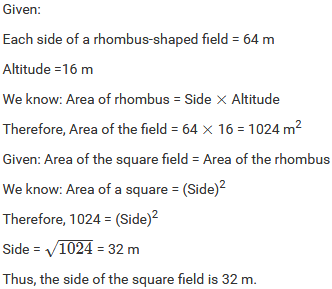Question: 22

The area a rhombus is equal to the area of a triangle whose base and the corresponding altitudes are 24.8 cm and 16.5 cm respectively. If one of the diagonals of the rhombus is 22 cm, find the length of the other diagonal.

Solution: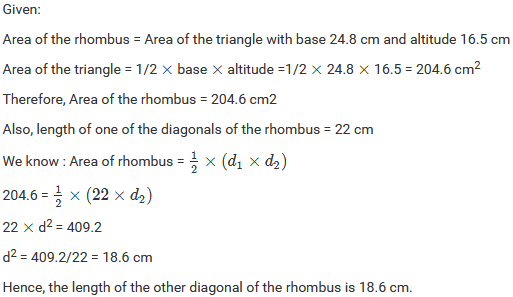### Course Features

• 728 Video Lectures
• Revision Notes
• Previous Year Papers
• Mind Map
• Study Planner
• NCERT Solutions
• Discussion Forum
• Test paper with Video Solution